## Is there any way to do cubic spline in Inquisit?

##### Is there any way to do cubic spline in Inquisit?
 Author Message Andrew Papale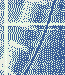posted 2 Months Ago ANSWER HOTGroup: Forum Members Posts: 58, Visits: 205 Dear Inquisit Community,Has anyone tried to implement a cubic spline algorithm through ~30 points in Inquisit?The problem: I have a list of values (e.g. 1-100) for each trial in an Inquisit list that roughly forms a continuous value landscape with peaks and valleys across 360 possible choices.  At some trial, I insert a new value (e.g. 25) into one of the 360 possible choices (say it was 100).  Therefore, the value at the point that used to be 100 is now 25.  Then, I want to fill in (as smoothly as possible) the values between the inserted value of 25 and the old value landscape that includes points adjacent to the old value (e.g. 90, 80, 70 on the left; and 110, 120, 130 on the right).Is anything like this even possible? I currently have a parabolic approximation through an endpoint and a vertex working fairly well, but thought I'd ask if there is a better solution.Thanks,Andrew Daveposted 2 Months Ago ANSWERGroup: Administrators Posts: 12K, Visits: 95K +x Andrew Papale - 10/26/2023Dear Inquisit Community,Has anyone tried to implement a cubic spline algorithm through ~30 points in Inquisit?The problem: I have a list of values (e.g. 1-100) for each trial in an Inquisit list that roughly forms a continuous value landscape with peaks and valleys across 360 possible choices.  At some trial, I insert a new value (e.g. 25) into one of the 360 possible choices (say it was 100).  Therefore, the value at the point that used to be 100 is now 25.  Then, I want to fill in (as smoothly as possible) the values between the inserted value of 25 and the old value landscape that includes points adjacent to the old value (e.g. 90, 80, 70 on the left; and 110, 120, 130 on the right).Is anything like this even possible? I currently have a parabolic approximation through an endpoint and a vertex working fairly well, but thought I'd ask if there is a better solution.Thanks,Andrew    For background see:    Press et al., Numerical Recipes in C: The Art of Scientific Computing, 2nd Ed. (1992),     Chapter 3.3 Cubic Spline Interpolation, pg. 113 - 116.// input: parameter space to construct the splines over// x values must be in ascending order, x values must be unique// items = (-10, -6.5, -3.5, -1.5, -0.2, 0, 1, 3.5, 5, 6.5, 10, 15, 20, 23, 30) // x values of 1st example input space / items = (-10, -6.5, -3.5, 0, 3.5, 6.5, 10) // x values of 2nd example input space // items = (-1.5, -0.2, 1.0, 5.0, 10.0, 15.0, 20.0) // x values of 3rd example input space // items = (0, 0.1, -0.1, -1.2, 0, -0.4, 0.5, -0.1, 0.1, 0, 2, 1, 5, 3, -1) // y values of 1st example input space / items = (0, 0.1, -0.1, 1, -0.1, 0.1, 0) // y values of 2nd example input space // items = (-1.2, 0.0, 0.5, 1.0, 1.2, 2.0, 1.0) // y values of 3rd example input space // temp storage// output y of 2nd derivative // constructs the cubic splines over the input parameter space/ createSpline = {    list.y2.reset();    list.u.reset();    var n = list.x.itemcount;    // setting up the vectors with n items    var i = 1;    while (i <= n) {        list.y2.appenditem(null);        list.u.appenditem(null);        i += 1;    };    i = 1;    // natural boundary condition on leftmost point    list.y2.setitem(0.0, i);    list.u.setitem(0.0, i);    // decomposition    i = 2;    while (i <= n-1) {        //sig=(x[i]-x[i-1])/(x[i+1]-x[i-1]);        var sig = (list.x.item(i) - list.x.item(i-1)) / (list.x.item(i+1) - list.x.item(i-1));        //p=sig*y2[i-1]+2.0        var p = sig * list.y2.item(i-1) + 2.0;        //y2[i]=(sig-1.0)/p;        var y2i = (sig - 1.0) / p;        list.y2.setitem(y2i, i);        //u[i]=(y[i+1]-y[i])/(x[i+1]-x[i]) - (y[i]-y[i-1])/(x[i]-x[i-1]);        var ui = (list.y.item(i+1) - list.y.item(i)) / (list.x.item(i+1) - list.x.item(i)) - (list.y.item(i) - list.y.item(i-1)) / (list.x.item(i) - list.x.item(i-1));        list.u.setitem(ui, i);        //u[i]=(6.0*u[i]/(x[i+1]-x[i-1])-sig*u[i-1])/p;        ui = (6.0 * list.u.item(i) / (list.x.item(i+1) - list.x.item(i-1)) - sig * list.u.item(i-1)) / p;        list.u.setitem(ui, i);        i += 1;    };    // natural boundary condition on rightmost point    var qn = 0.0;    var un = 0.0;    //y2[n]=(un-qn*u[n-1])/(qn*y2[n-1]+1.0);    var y2n = (un - qn * list.u.item(n-1)) / (qn * list.y2.item(n-1) + 1.0);    list.y2.setitem(y2n, n);    // backsubstitution    var k = n-1;    while (k >= 1) {        //y2[k]=y2[k]*y2[k+1]+u[k];        var y2k = list.y2.item(k) * list.y2.item(k+1) + list.u.item(k);        list.y2.setitem(y2k, k);        k -= 1;    };};// sets up a test vector of x values in the range x1 to xn/ createTestVector = {    var x1 = list.x.item(1);    var xn = list.x.item(list.x.itemcount);    var x = x1;    // set up a test vector of x values in range x1 to xn    while (x <= xn) {        list.x_test.appenditem(x);        list.y_test.appenditem(null);        x += 0.25; // decrease for denser sampling (more points), increase for sparser sampling (fewer points)    };};// given an x value, finds the insertion point and returns interpolated y value/ interpolateSpline = {    // bisect    var x = values.x;    var n = list.x.itemcount;    var klo = 1;    var khi = n;    while (khi - klo > 1) {        var k = floor((khi + klo) / 2); // same as right bit shift        if (list.x.item(k) > x) {            khi = k;        } else {            klo = k;        };    };    var h = list.x.item(khi) - list.x.item(klo);    //a=(xa[khi]-x)/h;    var a = (list.x.item(khi) - x) / h;    //b=(x-xa[klo])/h;    var b = (x - list.x.item(klo)) / h;    // calculate interpolated y    // y=a*ya[klo]+b*ya[khi]+((a*a*a-a)*y2a[klo]+(b*b*b-b)*y2a[khi])*(h*h)/6.0    var y = a * list.y.item(klo) + b * list.y.item(khi) + ((a*a*a - a) * list.y2.item(klo) + (b*b*b - b) * list.y2.item(khi)) * (h*h) / 6.0;    return y;};// test vectors holding interpolated x / y values / selectionmode = sequence/ x = null/ y = null/ onblockbegin = [    expressions.createSpline; // call the spline construction routine     expressions.createTestVector; // call the test vector generation routine]/ trials = [1=interpolateSpline]// pass x values to the interpolation routine, get interpolated y values/ ontrialbegin = [    values.x = list.x_test.nextvalue; // get our x value    values.y = expressions.interpolateSpline; // get our interpolated y value    list.y_test.setitem(values.y, list.x_test.currentindex); // add interpolated y to the test vector]/ trialduration = 0/ branch = [    if (list.x_test.selectedcount < list.x_test.itemcount) {    // run as often as we have x values in our test vector        return trial.interpolateSpline;                         // this is just for ease of data output and plotting, it's not a problem to     };                                                            // wrap everything in a single while() loop]/ columns = (trialnum values.x values.y) // write out x and y one value pair per row for easy plotting/ separatefiles = trueThe above is essentially an Inquisit implementation of the cubic spline interpolation algorithm outlined in Numerical Recipes in C.Plotting the output, i.e. interpolated x / y values, gives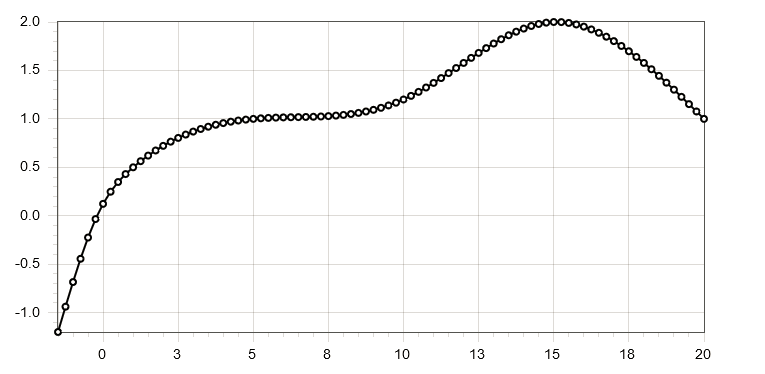with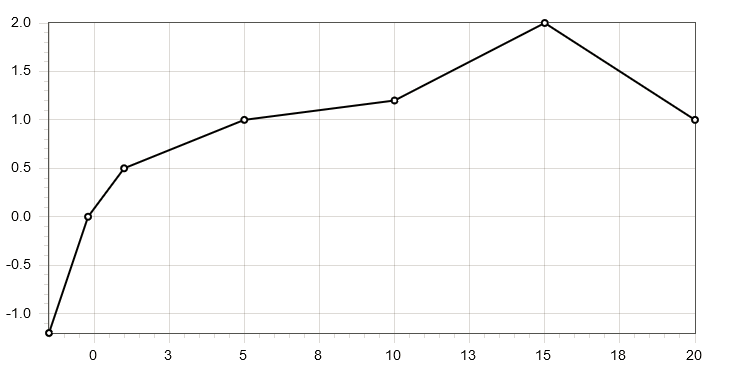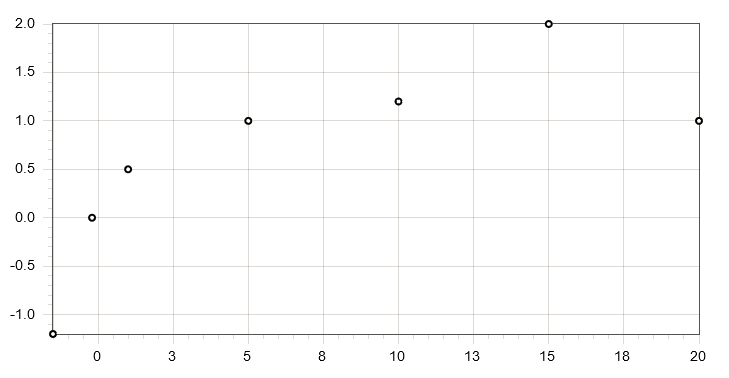being the original points the splines were constructed over (shown with & without linear interpolation).Or here's the interpolation result over a very different set of inputs/ items = (-10, -6.5, -3.5, 0, 3.5, 6.5, 10)/ items = (0, 0.1, -0.1, 1, -0.1, 0.1, 0)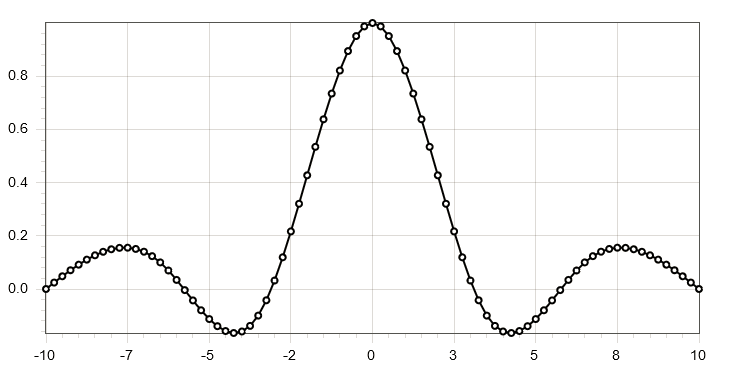Attachmentscubic_spline_numerical_recipes.iqx (27 views, 4.00 KB) Edited 2 Months Ago by Dave Andrew PapaleGroup: Forum Members Posts: 58, Visits: 205 +x Dave - 10/27/2023+x Andrew Papale - 10/26/2023Dear Inquisit Community,Has anyone tried to implement a cubic spline algorithm through ~30 points in Inquisit?The problem: I have a list of values (e.g. 1-100) for each trial in an Inquisit list that roughly forms a continuous value landscape with peaks and valleys across 360 possible choices.  At some trial, I insert a new value (e.g. 25) into one of the 360 possible choices (say it was 100).  Therefore, the value at the point that used to be 100 is now 25.  Then, I want to fill in (as smoothly as possible) the values between the inserted value of 25 and the old value landscape that includes points adjacent to the old value (e.g. 90, 80, 70 on the left; and 110, 120, 130 on the right).Is anything like this even possible? I currently have a parabolic approximation through an endpoint and a vertex working fairly well, but thought I'd ask if there is a better solution.Thanks,Andrew// input: parameter space to construct the splines over / items = (-1.5, -0.2, 1.0, 5.0, 10.0, 15.0, 20.0)/ items = (-1.2, 0.0, 0.5, 1.0, 1.2, 2.0, 1.0)// temp storage// output coefficients // constructs the cubic splines over the parameter space/ createSpline = {    list.y2.reset();    list.u.reset();    var n = list.x.itemcount;    // setting up the vectors with n items    var i = 1;    while (i <= n) {        list.y2.appenditem(null);        list.u.appenditem(null);        i += 1;    };    // set natural boundary condition    list.y2.setitem(0.0, 1);    list.u.setitem(0.0, 1);    // decomposition    i = 2;    while (i <= n-1) {        //sig=(x[i]-x[i-1])/(x[i+1]-x[i-1]);        var sig = (list.x.item(i) - list.x.item(i-1)) / (list.x.item(i+1) - list.x.item(i-1));        //p=sig*y2[i-1]+2.0        var p = sig * list.y2.item(i-1) + 2.0;        //y2[i]=(sig-1.0)/p;        list.y2.setitem((sig - 1.0) / p, i);        //u[i]=(y[i+1]-y[i])/(x[i+1]-x[i]) - (y[i]-y[i-1])/(x[i]-x[i-1]);        var ui = (list.y.item(i+1) - list.y.item(i)) / (list.x.item(i+1) - list.x.item(i)) - (list.y.item(i) - list.y.item(i-1)) / (list.x.item(i) - list.x.item(i-1));        list.u.setitem(ui, i);        //u[i]=(6.0*u[i]/(x[i+1]-x[i-1])-sig*u[i-1])/p;        ui = (6.0 * list.u.item(i) / (list.x.item(i+1) - list.x.item(i-1)) - sig * list.u.item(i-1)) / p;        list.u.setitem(ui, i);        i += 1;    };    // natural boundary condition    var qn = 0.0;    var un = 0.0;    //y2[n]=(un-qn*u[n-1])/(qn*y2[n-1]+1.0);    list.y2.setitem((un - qn * list.u.item(n-1)) / (qn * list.y2.item(n-1) + 1.0), n);    // backsubstitution    var k = n-1;    while (k >= 1) {        //y2[k]=y2[k]*y2[k+1]+u[k];        var y2k = list.y2.item(k) * list.y2.item(k+1) + list.u.item(k);        list.y2.setitem(y2k, k);        k -= 1;    };};// sets up a test vector of x values in the range x1 to xn/ createTestVector = {    var start_x = list.x.item(1);    var end_x = list.x.item(list.x.itemcount);    var x = start_x;    // set up a test vector of x values in range x1 to xn    while (x <= end_x) {        list.x_test.appenditem(x);        list.y_test.appenditem(null);        x += 0.25;    };};/ interpolateSpline = {    // bisect    var n = list.x.itemcount;    var klo = 1;    var khi = n;    while (khi - klo > 1) {        var k = floor((khi + klo) / 2); // same as right bit shift        if (list.x.item(k) > values.x) {            khi = k;        } else {            klo = k;        };    };    var h = list.x.item(khi) - list.x.item(klo);    //a=(xa[khi]-x)/h;    var a = (list.x.item(khi) - values.x) / h;    //b=(x-xa[klo])/h;    var b = (values.x - list.x.item(klo)) / h;    // calculate interpolated y    // y=a*ya[klo]+b*ya[khi]+((a*a*a-a)*y2a[klo]+(b*b*b-b)*y2a[khi])*(h*h)/6.0    values.y = a * list.y.item(klo) + b * list.y.item(khi) + ((a*a*a - a) * list.y2.item(klo) + (b*b*b -b) * list.y2.item(khi)) * (h*h) / 6.0;    return values.y;}; / selectionmode = sequence/ x = null/ y = null/ onblockbegin = [    expressions.createSpline;    expressions.createTestVector;]/ trials = [1=interpolateSpline]/ ontrialbegin = [    values.x = list.x_test.nextvalue; // get our x value    values.y = null;    expressions.interpolateSpline; // interpolate y    list.y_test.setitem(values.y, list.x_test.currentindex);]/ trialduration = 0/ branch = [    if (list.x_test.selectedcount < list.x_test.itemcount) {        return trial.interpolateSpline;    };]/ columns = (trialnum values.x values.y)/ separatefiles = trueThe above is essentially a straight Inquisit implementation of the cubic spline interpolation algorithm from Numerical Recipes in C.Plotting the output, i.e. interpolated x / y values, giveswithbeing the original points the splines were constructed over (shown with & without linear interpolation).Or here's the interpolation result over a very different set of inputs/ items = (-10, -6.5, -3.5, 0, 3.5, 6.5, 10)/ items = (0, 0.1, -0.1, 1, -0.1, 0.1, 0)Dave,This is absolutely fantastic.  Thank you so much on behalf of the entire lab.Andre Daveposted 2 Months Ago ANSWERGroup: Administrators Posts: 12K, Visits: 95K +x Andrew Papale - 10/28/2023+x Dave - 10/27/2023+x Andrew Papale - 10/26/2023Dear Inquisit Community,Has anyone tried to implement a cubic spline algorithm through ~30 points in Inquisit?The problem: I have a list of values (e.g. 1-100) for each trial in an Inquisit list that roughly forms a continuous value landscape with peaks and valleys across 360 possible choices.  At some trial, I insert a new value (e.g. 25) into one of the 360 possible choices (say it was 100).  Therefore, the value at the point that used to be 100 is now 25.  Then, I want to fill in (as smoothly as possible) the values between the inserted value of 25 and the old value landscape that includes points adjacent to the old value (e.g. 90, 80, 70 on the left; and 110, 120, 130 on the right).Is anything like this even possible? I currently have a parabolic approximation through an endpoint and a vertex working fairly well, but thought I'd ask if there is a better solution.Thanks,Andrew// input: parameter space to construct the splines over / items = (-1.5, -0.2, 1.0, 5.0, 10.0, 15.0, 20.0)/ items = (-1.2, 0.0, 0.5, 1.0, 1.2, 2.0, 1.0)// temp storage// output coefficients // constructs the cubic splines over the parameter space/ createSpline = {    list.y2.reset();    list.u.reset();    var n = list.x.itemcount;    // setting up the vectors with n items    var i = 1;    while (i <= n) {        list.y2.appenditem(null);        list.u.appenditem(null);        i += 1;    };    // set natural boundary condition    list.y2.setitem(0.0, 1);    list.u.setitem(0.0, 1);    // decomposition    i = 2;    while (i <= n-1) {        //sig=(x[i]-x[i-1])/(x[i+1]-x[i-1]);        var sig = (list.x.item(i) - list.x.item(i-1)) / (list.x.item(i+1) - list.x.item(i-1));        //p=sig*y2[i-1]+2.0        var p = sig * list.y2.item(i-1) + 2.0;        //y2[i]=(sig-1.0)/p;        list.y2.setitem((sig - 1.0) / p, i);        //u[i]=(y[i+1]-y[i])/(x[i+1]-x[i]) - (y[i]-y[i-1])/(x[i]-x[i-1]);        var ui = (list.y.item(i+1) - list.y.item(i)) / (list.x.item(i+1) - list.x.item(i)) - (list.y.item(i) - list.y.item(i-1)) / (list.x.item(i) - list.x.item(i-1));        list.u.setitem(ui, i);        //u[i]=(6.0*u[i]/(x[i+1]-x[i-1])-sig*u[i-1])/p;        ui = (6.0 * list.u.item(i) / (list.x.item(i+1) - list.x.item(i-1)) - sig * list.u.item(i-1)) / p;        list.u.setitem(ui, i);        i += 1;    };    // natural boundary condition    var qn = 0.0;    var un = 0.0;    //y2[n]=(un-qn*u[n-1])/(qn*y2[n-1]+1.0);    list.y2.setitem((un - qn * list.u.item(n-1)) / (qn * list.y2.item(n-1) + 1.0), n);    // backsubstitution    var k = n-1;    while (k >= 1) {        //y2[k]=y2[k]*y2[k+1]+u[k];        var y2k = list.y2.item(k) * list.y2.item(k+1) + list.u.item(k);        list.y2.setitem(y2k, k);        k -= 1;    };};// sets up a test vector of x values in the range x1 to xn/ createTestVector = {    var start_x = list.x.item(1);    var end_x = list.x.item(list.x.itemcount);    var x = start_x;    // set up a test vector of x values in range x1 to xn    while (x <= end_x) {        list.x_test.appenditem(x);        list.y_test.appenditem(null);        x += 0.25;    };};/ interpolateSpline = {    // bisect    var n = list.x.itemcount;    var klo = 1;    var khi = n;    while (khi - klo > 1) {        var k = floor((khi + klo) / 2); // same as right bit shift        if (list.x.item(k) > values.x) {            khi = k;        } else {            klo = k;        };    };    var h = list.x.item(khi) - list.x.item(klo);    //a=(xa[khi]-x)/h;    var a = (list.x.item(khi) - values.x) / h;    //b=(x-xa[klo])/h;    var b = (values.x - list.x.item(klo)) / h;    // calculate interpolated y    // y=a*ya[klo]+b*ya[khi]+((a*a*a-a)*y2a[klo]+(b*b*b-b)*y2a[khi])*(h*h)/6.0    values.y = a * list.y.item(klo) + b * list.y.item(khi) + ((a*a*a - a) * list.y2.item(klo) + (b*b*b -b) * list.y2.item(khi)) * (h*h) / 6.0;    return values.y;}; / selectionmode = sequence/ x = null/ y = null/ onblockbegin = [    expressions.createSpline;    expressions.createTestVector;]/ trials = [1=interpolateSpline]/ ontrialbegin = [    values.x = list.x_test.nextvalue; // get our x value    values.y = null;    expressions.interpolateSpline; // interpolate y    list.y_test.setitem(values.y, list.x_test.currentindex);]/ trialduration = 0/ branch = [    if (list.x_test.selectedcount < list.x_test.itemcount) {        return trial.interpolateSpline;    };]/ columns = (trialnum values.x values.y)/ separatefiles = trueThe above is essentially a straight Inquisit implementation of the cubic spline interpolation algorithm from Numerical Recipes in C.Plotting the output, i.e. interpolated x / y values, giveswithbeing the original points the splines were constructed over (shown with & without linear interpolation).Or here's the interpolation result over a very different set of inputs/ items = (-10, -6.5, -3.5, 0, 3.5, 6.5, 10)/ items = (0, 0.1, -0.1, 1, -0.1, 0.1, 0)Dave,This is absolutely fantastic.  Thank you so much on behalf of the entire lab.AndreFYI, I cleaned up the code in the original response a little bit and added a few more test inputs and comments. (No bug fixes or major changes to the code itself, so it's fine to keep using the previous version.) Andrew PapaleGroup: Forum Members Posts: 58, Visits: 205 +x Dave - 10/30/2023+x Andrew Papale - 10/28/2023+x Dave - 10/27/2023+x Andrew Papale - 10/26/2023Dear Inquisit Community,Has anyone tried to implement a cubic spline algorithm through ~30 points in Inquisit?The problem: I have a list of values (e.g. 1-100) for each trial in an Inquisit list that roughly forms a continuous value landscape with peaks and valleys across 360 possible choices.  At some trial, I insert a new value (e.g. 25) into one of the 360 possible choices (say it was 100).  Therefore, the value at the point that used to be 100 is now 25.  Then, I want to fill in (as smoothly as possible) the values between the inserted value of 25 and the old value landscape that includes points adjacent to the old value (e.g. 90, 80, 70 on the left; and 110, 120, 130 on the right).Is anything like this even possible? I currently have a parabolic approximation through an endpoint and a vertex working fairly well, but thought I'd ask if there is a better solution.Thanks,Andrew// input: parameter space to construct the splines over / items = (-1.5, -0.2, 1.0, 5.0, 10.0, 15.0, 20.0)/ items = (-1.2, 0.0, 0.5, 1.0, 1.2, 2.0, 1.0)// temp storage// output coefficients // constructs the cubic splines over the parameter space/ createSpline = {    list.y2.reset();    list.u.reset();    var n = list.x.itemcount;    // setting up the vectors with n items    var i = 1;    while (i <= n) {        list.y2.appenditem(null);        list.u.appenditem(null);        i += 1;    };    // set natural boundary condition    list.y2.setitem(0.0, 1);    list.u.setitem(0.0, 1);    // decomposition    i = 2;    while (i <= n-1) {        //sig=(x[i]-x[i-1])/(x[i+1]-x[i-1]);        var sig = (list.x.item(i) - list.x.item(i-1)) / (list.x.item(i+1) - list.x.item(i-1));        //p=sig*y2[i-1]+2.0        var p = sig * list.y2.item(i-1) + 2.0;        //y2[i]=(sig-1.0)/p;        list.y2.setitem((sig - 1.0) / p, i);        //u[i]=(y[i+1]-y[i])/(x[i+1]-x[i]) - (y[i]-y[i-1])/(x[i]-x[i-1]);        var ui = (list.y.item(i+1) - list.y.item(i)) / (list.x.item(i+1) - list.x.item(i)) - (list.y.item(i) - list.y.item(i-1)) / (list.x.item(i) - list.x.item(i-1));        list.u.setitem(ui, i);        //u[i]=(6.0*u[i]/(x[i+1]-x[i-1])-sig*u[i-1])/p;        ui = (6.0 * list.u.item(i) / (list.x.item(i+1) - list.x.item(i-1)) - sig * list.u.item(i-1)) / p;        list.u.setitem(ui, i);        i += 1;    };    // natural boundary condition    var qn = 0.0;    var un = 0.0;    //y2[n]=(un-qn*u[n-1])/(qn*y2[n-1]+1.0);    list.y2.setitem((un - qn * list.u.item(n-1)) / (qn * list.y2.item(n-1) + 1.0), n);    // backsubstitution    var k = n-1;    while (k >= 1) {        //y2[k]=y2[k]*y2[k+1]+u[k];        var y2k = list.y2.item(k) * list.y2.item(k+1) + list.u.item(k);        list.y2.setitem(y2k, k);        k -= 1;    };};// sets up a test vector of x values in the range x1 to xn/ createTestVector = {    var start_x = list.x.item(1);    var end_x = list.x.item(list.x.itemcount);    var x = start_x;    // set up a test vector of x values in range x1 to xn    while (x <= end_x) {        list.x_test.appenditem(x);        list.y_test.appenditem(null);        x += 0.25;    };};/ interpolateSpline = {    // bisect    var n = list.x.itemcount;    var klo = 1;    var khi = n;    while (khi - klo > 1) {        var k = floor((khi + klo) / 2); // same as right bit shift        if (list.x.item(k) > values.x) {            khi = k;        } else {            klo = k;        };    };    var h = list.x.item(khi) - list.x.item(klo);    //a=(xa[khi]-x)/h;    var a = (list.x.item(khi) - values.x) / h;    //b=(x-xa[klo])/h;    var b = (values.x - list.x.item(klo)) / h;    // calculate interpolated y    // y=a*ya[klo]+b*ya[khi]+((a*a*a-a)*y2a[klo]+(b*b*b-b)*y2a[khi])*(h*h)/6.0    values.y = a * list.y.item(klo) + b * list.y.item(khi) + ((a*a*a - a) * list.y2.item(klo) + (b*b*b -b) * list.y2.item(khi)) * (h*h) / 6.0;    return values.y;}; / selectionmode = sequence/ x = null/ y = null/ onblockbegin = [    expressions.createSpline;    expressions.createTestVector;]/ trials = [1=interpolateSpline]/ ontrialbegin = [    values.x = list.x_test.nextvalue; // get our x value    values.y = null;    expressions.interpolateSpline; // interpolate y    list.y_test.setitem(values.y, list.x_test.currentindex);]/ trialduration = 0/ branch = [    if (list.x_test.selectedcount < list.x_test.itemcount) {        return trial.interpolateSpline;    };]/ columns = (trialnum values.x values.y)/ separatefiles = trueThe above is essentially a straight Inquisit implementation of the cubic spline interpolation algorithm from Numerical Recipes in C.Plotting the output, i.e. interpolated x / y values, giveswithbeing the original points the splines were constructed over (shown with & without linear interpolation).Or here's the interpolation result over a very different set of inputs/ items = (-10, -6.5, -3.5, 0, 3.5, 6.5, 10)/ items = (0, 0.1, -0.1, 1, -0.1, 0.1, 0)Dave,This is absolutely fantastic.  Thank you so much on behalf of the entire lab.AndreFYI, I cleaned up the code in the original response a little bit and added a few more test inputs and comments. (No bug fixes or major changes to the code itself, so it's fine to keep using the previous version.)Thanks Dave, I've got this working basically how we want it to now (see attached).  We have these values mapped onto 360 degrees of a circle and I need to figure out 1) how to handle the cases where these insertions appear on the edges near 0/360 degrees.  Then, I need to 2) iteratively insert all these erasures (which will occur at some frequency set by the experimental design) into all future trials, which occur in multiples of 360 in the values-5888.txt and RTs-5888.txt vectors.  I have a general idea of how to proceed with 2) using while loops and modular division and 1) shifting the RT values, performing the spline interpolation, and then shifting them back into place. Any advice is appreciated, though I feel like you've done enough for us here already.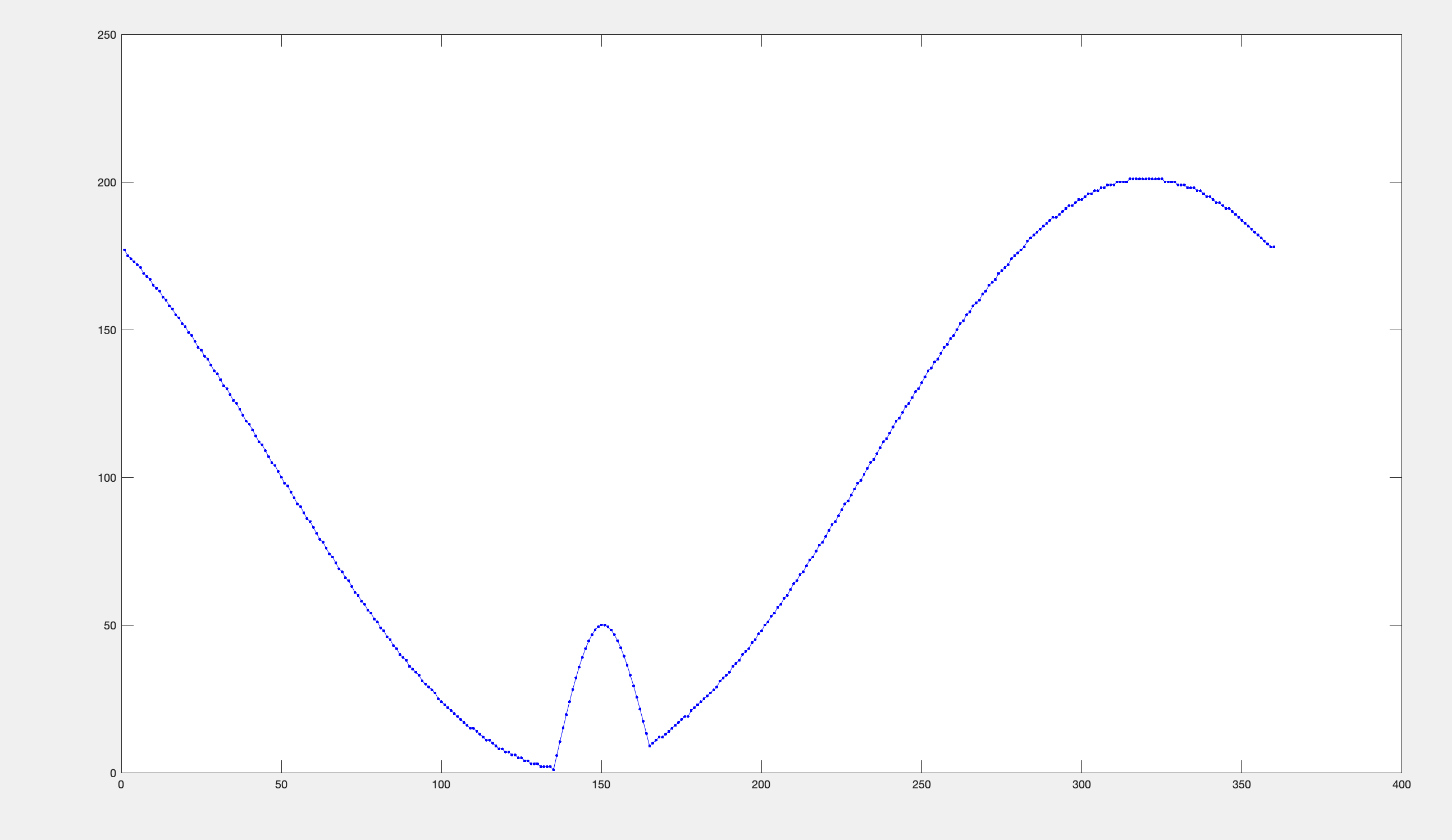Attachmentstest_spline.iqx (26 views, 7.00 KB)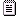RTs-5888.txt (28 views, 390.00 KB)values-5888.txt (27 views, 324.00 KB) Andrew PapaleGroup: Forum Members Posts: 58, Visits: 205 +x Andrew Papale - 11/1/2023+x Dave - 10/30/2023+x Andrew Papale - 10/28/2023+x Dave - 10/27/2023+x Andrew Papale - 10/26/2023Dear Inquisit Community,Has anyone tried to implement a cubic spline algorithm through ~30 points in Inquisit?The problem: I have a list of values (e.g. 1-100) for each trial in an Inquisit list that roughly forms a continuous value landscape with peaks and valleys across 360 possible choices.  At some trial, I insert a new value (e.g. 25) into one of the 360 possible choices (say it was 100).  Therefore, the value at the point that used to be 100 is now 25.  Then, I want to fill in (as smoothly as possible) the values between the inserted value of 25 and the old value landscape that includes points adjacent to the old value (e.g. 90, 80, 70 on the left; and 110, 120, 130 on the right).Is anything like this even possible? I currently have a parabolic approximation through an endpoint and a vertex working fairly well, but thought I'd ask if there is a better solution.Thanks,Andrew// input: parameter space to construct the splines over / items = (-1.5, -0.2, 1.0, 5.0, 10.0, 15.0, 20.0)/ items = (-1.2, 0.0, 0.5, 1.0, 1.2, 2.0, 1.0)// temp storage// output coefficients // constructs the cubic splines over the parameter space/ createSpline = {    list.y2.reset();    list.u.reset();    var n = list.x.itemcount;    // setting up the vectors with n items    var i = 1;    while (i <= n) {        list.y2.appenditem(null);        list.u.appenditem(null);        i += 1;    };    // set natural boundary condition    list.y2.setitem(0.0, 1);    list.u.setitem(0.0, 1);    // decomposition    i = 2;    while (i <= n-1) {        //sig=(x[i]-x[i-1])/(x[i+1]-x[i-1]);        var sig = (list.x.item(i) - list.x.item(i-1)) / (list.x.item(i+1) - list.x.item(i-1));        //p=sig*y2[i-1]+2.0        var p = sig * list.y2.item(i-1) + 2.0;        //y2[i]=(sig-1.0)/p;        list.y2.setitem((sig - 1.0) / p, i);        //u[i]=(y[i+1]-y[i])/(x[i+1]-x[i]) - (y[i]-y[i-1])/(x[i]-x[i-1]);        var ui = (list.y.item(i+1) - list.y.item(i)) / (list.x.item(i+1) - list.x.item(i)) - (list.y.item(i) - list.y.item(i-1)) / (list.x.item(i) - list.x.item(i-1));        list.u.setitem(ui, i);        //u[i]=(6.0*u[i]/(x[i+1]-x[i-1])-sig*u[i-1])/p;        ui = (6.0 * list.u.item(i) / (list.x.item(i+1) - list.x.item(i-1)) - sig * list.u.item(i-1)) / p;        list.u.setitem(ui, i);        i += 1;    };    // natural boundary condition    var qn = 0.0;    var un = 0.0;    //y2[n]=(un-qn*u[n-1])/(qn*y2[n-1]+1.0);    list.y2.setitem((un - qn * list.u.item(n-1)) / (qn * list.y2.item(n-1) + 1.0), n);    // backsubstitution    var k = n-1;    while (k >= 1) {        //y2[k]=y2[k]*y2[k+1]+u[k];        var y2k = list.y2.item(k) * list.y2.item(k+1) + list.u.item(k);        list.y2.setitem(y2k, k);        k -= 1;    };};// sets up a test vector of x values in the range x1 to xn/ createTestVector = {    var start_x = list.x.item(1);    var end_x = list.x.item(list.x.itemcount);    var x = start_x;    // set up a test vector of x values in range x1 to xn    while (x <= end_x) {        list.x_test.appenditem(x);        list.y_test.appenditem(null);        x += 0.25;    };};/ interpolateSpline = {    // bisect    var n = list.x.itemcount;    var klo = 1;    var khi = n;    while (khi - klo > 1) {        var k = floor((khi + klo) / 2); // same as right bit shift        if (list.x.item(k) > values.x) {            khi = k;        } else {            klo = k;        };    };    var h = list.x.item(khi) - list.x.item(klo);    //a=(xa[khi]-x)/h;    var a = (list.x.item(khi) - values.x) / h;    //b=(x-xa[klo])/h;    var b = (values.x - list.x.item(klo)) / h;    // calculate interpolated y    // y=a*ya[klo]+b*ya[khi]+((a*a*a-a)*y2a[klo]+(b*b*b-b)*y2a[khi])*(h*h)/6.0    values.y = a * list.y.item(klo) + b * list.y.item(khi) + ((a*a*a - a) * list.y2.item(klo) + (b*b*b -b) * list.y2.item(khi)) * (h*h) / 6.0;    return values.y;}; / selectionmode = sequence/ x = null/ y = null/ onblockbegin = [    expressions.createSpline;    expressions.createTestVector;]/ trials = [1=interpolateSpline]/ ontrialbegin = [    values.x = list.x_test.nextvalue; // get our x value    values.y = null;    expressions.interpolateSpline; // interpolate y    list.y_test.setitem(values.y, list.x_test.currentindex);]/ trialduration = 0/ branch = [    if (list.x_test.selectedcount < list.x_test.itemcount) {        return trial.interpolateSpline;    };]/ columns = (trialnum values.x values.y)/ separatefiles = trueThe above is essentially a straight Inquisit implementation of the cubic spline interpolation algorithm from Numerical Recipes in C.Plotting the output, i.e. interpolated x / y values, giveswith